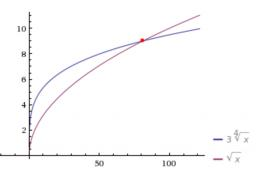# One-fourth 3820

Darius said to Miloš:

I think a number. If I square it to one-fourth and then multiply it by 3, I get its square root. Find the unknown number that is greater than zero and belongs to the set of positive integers.

x =  81

### Step-by-step explanation:Did you find an error or inaccuracy? Feel free to write us. Thank you!

Tips for related online calculators
Do you have a linear equation or system of equations and looking for its solution? Or do you have a quadratic equation?